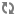Apache NiFi Configuration Best Practices# Analytics Framework

NiFi has an internal analytics framework which can be enabled to predict back pressure occurrence, given the configured settings for threshold on a queue. The model used by default for prediction is an ordinary least squares (OLS) linear regression. It uses recent observations from a queue (either number of objects or content size over time) and calculates a regression line for that data. The line's equation is then used to determine the next value that will be reached within a given time interval (e.g. number of objects in queue in the next 5 minutes). Below is an example graph of the linear regression model for Queue/Object Count over time which is used for predictions:In order to generate predictions, local status snapshot history is queried to obtain enough data to generate a model. By default, component status snapshots are captured every minute. Internal models need at least 2 or more observations to generate a prediction, therefore it may take up to 2 or more minutes for predictions to be available by default. If predictions are needed sooner than what is provided by default, the timing of snapshots can be adjusted using the `nifi.components.status.snapshot.frequency` value in nifi.properties.

NiFi evaluates the model's effectiveness before sending prediction information by using the model's R-Squared score by default. One important note: R-Square is a measure of how close the regression line fits the observation data vs. how accurate the prediction will be; therefore there may be some measure of error. If the R-Squared score for the calculated model meets the configured threshold (as defined by `nifi.analytics.connection.model.score.threshold`) then the model will be used for prediction. Otherwise the model will not be used and predictions will not be available until a model is generated with a score that exceeds the threshold. Default R-Squared threshold value is `.90` however this can be tuned based on prediction requirements.

The prediction interval `nifi.analytics.predict.interval` can be configured to project out further when back pressure will occur. The prediction query interval `nifi.analytics.query.interval` can also be configured to determine how far back in time past observations should be queried in order to generate the model. Adjustments to these settings may require tuning of the model's scoring threshold value to select a score that can offer reasonable predictions.

See Analytics Properties for complete information on configuring analytic properties.